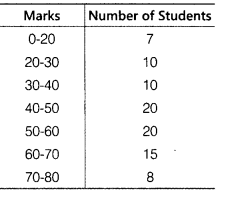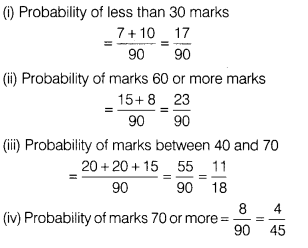# Following table shows the marks scored by a group of 90 students in a mathematics test of 100

Following table shows the marks scored by a group of 90 students in a mathematics test of 100 marks.A student is selected at random. Find the probability that student has obtained.
(i) Less than 30.
(ii) 60 or more marks.
(iii) between 40 and 70 marks.
(iv) 70 or more marks